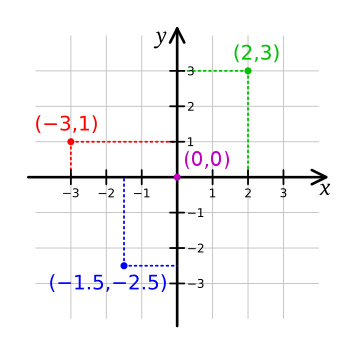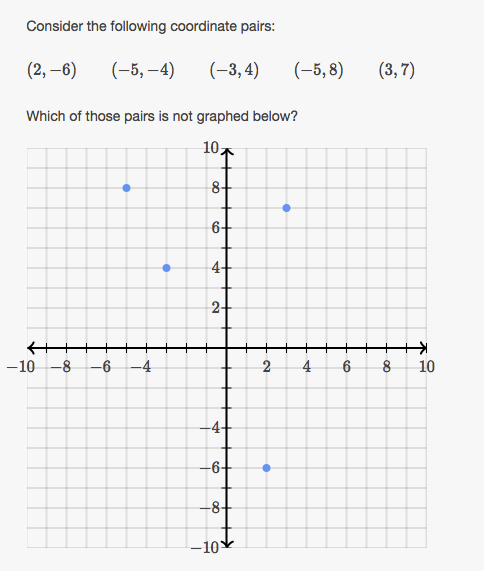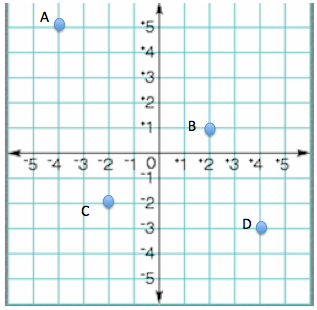# Coordinate System

coordinate plane is formed by two number lines in a plane that intersect at right angles. The two number lines intersect at 0 on each number line and this point of intersection is called the origin. The two number lines are called axes. The horizontal axis is called the x-axis. The vertical axis is called the y-axis. The coordinate plane is divided into 4 sections called quadrants.An ordered pair is a pair of numbers (x, y) that indicate the position of a point on the Cartesian Plane. The numbers in an ordered pair are called coordinates.  The first number is called the x-coordinate and the second number is called the y-coordinate.   The x-coordinate tells how far to move right (positive) or left (negative) from the origin.   The y-coordinate tells how far to move up (positive) or down (negative) from the origin.   The ordered pair for the origin is (0, 0).

Let’s graph the ordered pair (1, 2). x=1, so begin at the origin and count over 1 unit to the right along the x-axis and draw the point. y=2, so count up two units along the y-axis and draw the point.

Just remember, x comes before y in the alphabet, so you will always move along the x-axis first.

If you aren’t sure on how to plot points, here’s a video.

## Practice

1.2.3. What are the coordinates of each point?(source)